### Home > CALC > Chapter 1 > Lesson 1.2.3 > Problem1-59

1-59.
1. Each of the continuous functions in the table below is increasing, but each increases differently. Match each graph below with the function that grows in a similar fashion in the table. 1-59 HW eTool (Desmos). Homework Help ✎

x

1

2

3

4

5

6

7

8

9

f(x)

64

68.8

74.6

81.5

89.8

99.7

111.7

126

143.2

g(x)

38

52

66

80

94

108

122

136

150

h(x)

22

42.9

57.3

68.5

77.6

85.3

92

97.9

103.1

 a.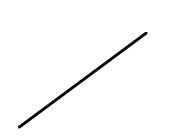b.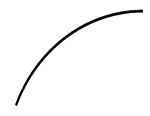c.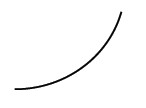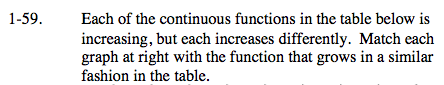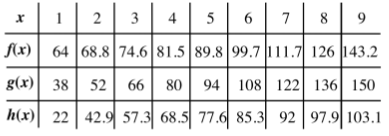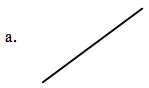Graph (a) is linear. Straight lines grow at a constant rate. Which function on the table is growing at a constant rate?

$\text{If rate of change is constant, then }\frac{\Delta y}{\Delta x}\text{ will be constant.}$

$\text{Compute }\frac{\Delta y}{\Delta x}\text{ for the first few values of }f(x):$

$\frac{68.8-64}{2-1}=4.4 {\ \ \ \ \ \ \ \ \ }\frac{74.6-68.8}{3-2}=5.8$

Since 4.4 ≠ 5.8, f(x) does not change at a constant rate. Try g(x) and h(x)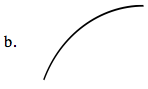Graph (b) is increasing (the y-values are going up), but it is getting less and less steep as it increases.

$\text{Steepness can be determined by rate of change: }\frac{\Delta y}{\Delta x}$

Which function on the table is getting less and less steep?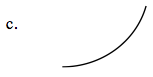Graph (c) is increasing (the y-values are going up), AND it is getting more and more steep as it increases.

Use the eTool below to create the graphs of the functions.
Click the link at right for the full version of the eTool: Calc 1-59 HW eTool